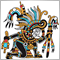# Maximum range candle of last 5 candles106

Hello,

I want to identify the candle (in the last 5) that have maximum range (High[i]-Low[i]) .

I wrote this code but for fill an array with range size:

```int candele = candelePrecedentiBuy;

int i = 1;

while (i <= candele) {

RangeCandela[i] = High - Low ;

}  ```

How can I retrieve the index in the array with maximum value?

I suppose to use ArrayMax fuction, is correct:

`int MaxRangeIndex = ArrayMaximum(RangeCandela);`

Thanks for help

Giovanni35094

```int index=0;
double delta=0;

for(int i=0;i<5;i++)
{
if (delta<High[i]-Low[i])
{
delta=High[i]-Low[i];
index=i;
}
}```